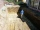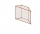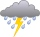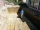# Prism Problems

A prism is a polyhedron comprising an n-sided polygonal base, a second base which is a translated copy (rigidly moved without rotation) of the first, and n other faces (necessarily all parallelograms) joining corresponding sides of the two bases. All cross-sections parallel to the bases are translations of the bases. Prisms are named for their bases, so a prism with a pentagonal base is called a pentagonal prism.

#### Number of problems found: 228

• Roof repairTo repair the roof, we need 15 pieces of boards 6 m long, 15 cm wide and 25 mm thick. How many euros will we pay for all boards if 1 m3 of boards costs 500 euros?
• The bodyThe body has dimensions of 2m, 2dm, and 10 cm. It weighs 28 kg. What is its density?
• Brick wallWhat is the weight of a solid brick wall that is 30 cm wide, 4 m long and 2 m high? The density of the brick is 1500 kg per cubic meter.
• Wooden blockA cuboid-shaped wooden block has 6 cm length, 4 cm breadth, and 1 cm height. Two faces measuring 4 cm x 1 cm are colored in black. Two faces measuring 6 cm x 1 cm are colored in red. Two faces measuring 6 cm x 4 cm are colored in green. The block is divid
• Edge of prismThe regular quadrilateral prism has a surface of 250 dm2, its shell has a content of 200 dm2. Calculate its leading edge.
• The trenchCalculate how many cubic meters of soil needs to be removed from the excavation in the shape of an isosceles trapezoid, the top width is 3 meters, the lower width is 1.8 m, the depth of the excavation is 1 m, and the length is 20 m.
• Largest wallFind the content of the largest wall of a prism with a rectangle base with a height of 4 dm, side c = 5 cm, and side b = 6 cm.Calculate the surface of a quadrilateral prism according to the input: Area of the diamond base S1 = 2.8 m2, length of the base edge a = 14 dm, height of the prism 1,500 mm.The surface of the regular quadrilateral prism is 8800 cm2, the base edge is 20 cm long. Calculate the volume of the prism
• Trapezoidal prismCalculate the volume of a prism with a trapezoidal base with side a = 6dm, side c = 4 dm, height of the prism = 8dm. The height of the trapezoid is va = 3dm.
• Triangular prismCalculate the surface of a regular triangular prism, the edges of the base are 6 cm long and the height of the prism is 15 cm.
• Cutting the prismA prism with a square base with a content of 1 cm2 and a height of 3 cm was cut from a cube with an edge length of 3 cm. What is the surface of the body formed from the cube after cutting the prism?
• Identical cubesFrom the smallest number of identical cubes whose edge length is expressed by a natural number, can we build a block with dimensions 12dm x 16dm x 20dm?
• Water tank300hl of water was filled into the tank 12 m long and 6 m wide. How high does it reach?
• RainGarden shape of a rectangle measuring 15 m and 20 m rained water up to 3 mm. How many liters of water rained in the garden?
• Wall and body diagonalsThe block/cuboid has dimensions a = 4cm, b = 3cm and c = 12cm. Calculates the length of the wall and body diagonals.
• EmbankmentThe railway embankment 300 m long has a cross-section of an isosceles trapezoid with bases of 14 m and 8 m. The trapezoidal arms are 5 m long. Calculate how much m3 of soil is in the embankment?
• Fire tank1428 hl of water is filled in a block-shaped fire tank with the edges of the base 12 m and 7 m. Calculate the content of water-wetted areas.
• The excavationThe excavation for the city sewer was 38 m long, 2.2 m wide, and 3 m deep. a) How many cubic meters of soil were dredged b) How many journeys had to be made by one car when removing the soil if they loaded an average of 4.5 m of cubic soil on each journey
• Triangular prismThe base of the perpendicular triangular prism is a rectangular triangle with a hypotenuse of 10 cm and one leg of 8 cm. The prism height is 75% of the perimeter of the base. Calculate the volume and surface of the prism.

Do you have an interesting mathematical word problem that you can't solve? Submit a math problem, and we can try to solve it.

We will send a solution to your e-mail address. Solved examples are also published here. Please enter the e-mail correctly and check whether you don't have a full mailbox.

Please do not submit problems from current active competitions such as Mathematical Olympiad, correspondence seminars etc...